### Home > AC > Chapter 5 > Lesson 5.1.3 > Problem5-30

5-30.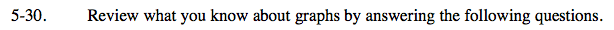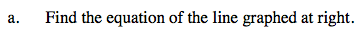Use the y-intercept and the growth rate to write the equation of the line at right.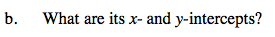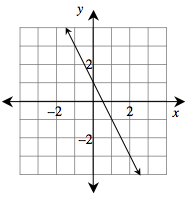Remember that the x-intercept is the point on the graph where y = 0 and that the y-intercept is the point where x = 0.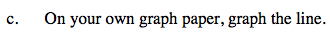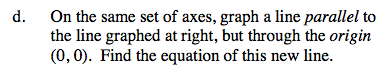Remember that parallel lines have the exact same growth rate.

Plug in the point given (0,0) into the basic equation y = mx + b and simplify to find the equation of the line.

m = the growth rate
b = the y-intercept